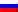# Backpack problem

Contenus

## Backpack problem

The backpack problem is one of Richard Karp's 21 NP-Complete Problems, outlined in his 1972 article.

# States

The backpack problem models a situation analogous to the filling of a backpack, which can no longer support a certain weight, with all or part of a given set of objects each having a weight and a value. The items put in the backpack must maximize the total value, without exceeding the maximum weight.

The most common form is the 0-1 (0-1 KP) backpack problem, restricting the number of copies xi of each object i to a maximum of one. Let be a set of n object, endowed with a weight wi and of utility vi, and the capacity of the backpack W, the problem is written as follows:

maximize ∑i = 1not vi* xi
subject to ∑i = 1not vi* xi ≤ W and xi ∈ {0.1}.

The bounded knapsack problem BKP removes the restriction of limiting the object by a limitation bounded by an integer ci:

maximize ∑i = 1not vi* xi
subject to ∑i = 1not vi* xi ≤ W and xi ∈ {0,…, ci}.

The unbounded knapsack problem UKP does not place any restrictions on the number of copies of each item.

maximize ∑i = 1not vi* xi
subject to ∑i = 1not vi* xi ≤ W and 0≤xi .There are many other variations of the backpack problem which are described on another page. Please refer to Polynomial Reduction.

# Resolution methods

## Greedy algorithm

The idea is to add the most effective objects as a priority, until the bag is saturated:

``` Sort the objects in decreasing order of efficiency w_conso: = 0

for i of 1 To not
if w [i] + w_conso ≤ W so
x [i]: = 1 w_conso: = w_conso + w [i]
otherwise
x [i]: = 0
end if
end for
```

## Dynamic programming

The backpack problem has the property of optimal substructure, ie that we can build the optimal solution of the k-variable problem from the k - 1-variable problem.

Let Fk(E) the best cost to fill a size E bag with the k
first objects. The recurrence shows that Fk(E) = max {Fk − 1(E), Fk − 1(E - wk) + vk},

indeed the addition of a new object increases or not the best cost. If the new object is not added to the bag, Fk(E) is equal to Fk − 1(E). In the case where the new object offers a better utility, ie in the case where the object is not present but the bag (without the object) has sufficient space to accommodate the new element, and it is optimal (i.e. the formula Fk − 1(E - wk)), then Fk(E) is equal to Fk − 1(E - wk) + vk, vk being the usefulness of the new object added to the bag described above.

We therefore seek to calculate Fnot(W), with n the total number of objects and W the maximum size of the backpack studied.

```for vs of 0 To W to do
T [0, c]: = 0
end for

for i of 1 To not to do
for vs of 0 To W to do
if c> = w [i] so
T [i, c]: = max (T [i-1, c], T [i-1, cw [i]] + p [i])
otherwise
T [i, c]: = T [i-1, c]
end if
end for
end for ```

We have the following elements: C = 8, and five objects described in
the table below

 i 1 2 3 4 5 p_i 3 4 5 4 2 u_i 15 18 20 12 6

We get the following backpack result table:

 E | i 1 2 3 4 5 0 0 0 0 0 0 1 0 0 0 0 0 2 6 6 6 6 6 3 15 6 6 6 6 4 15 18 12 12 6 5 21 20 20 12 6 6 24 24 20 18 6 7 33 26 26 18 6 8 35 30 26 18 6

The optimal solution of 25 is obtained with elements 1 and 3 according to the second
backpack algorithm:

 k 1 2 3 4 5 E 8 5 5 0 0 x [k] 1 0 1 0 0

conso = conso_1 + conso_3 = 8.FRFRENRUES